# Exhaustion, method of

A method of proof used by mathematicians of antiquity in order to determine areas and volumes. The name "method of exhaustion" was introduced in the 17th century.

The typical scheme of proof by the method of exhaustion can, in modern terms, be explained as follows. In order to determine a quantityone constructs a certain sequence of quantities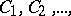such that(1)

one assumes that ais known such that(2)

and that for any integerand all sufficiently largethe inequalities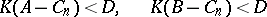(3)

are fulfilled, witha constant. From the modern point of view, to transfer (3) to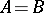(4)

one only has to notice that (1)–(3) imply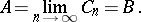The mathematicians of antiquity, not having developed the theory of limits (cf. Limit), used a reductio ad absurdum argument here: they proved that neither of the inequalities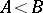,is possible. To disprove the first one, they established by the Archimedean axiom that forthere exists asuch that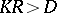, and (1) then led to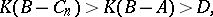which contradicts the second inequality in (3). The other assertion is disproved in a similar way. Hence (4) remains.

The introduction of the method of exhaustion and of the axiom that lies at its foundation is ascribed to Eudoxus of Cnidus. The method was extensively used by Eudoxus, while Archimedes used it with extraordinary skill and variety. E.g., in order to determine the areaof a segment of a parabola, Archimedes constructs the areasof segments that are stepwise "exhausting" the area.

Here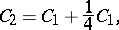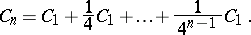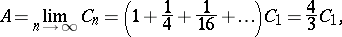Archimedes proves geometrically that for any,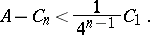Introducing the area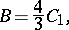he obtainsand, following the reasoning explained above, finishes his proof with